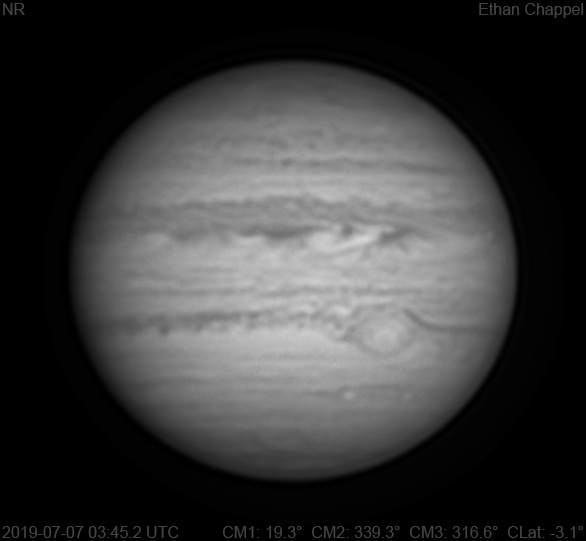# Jupiter 2019-07-07 03:45 UTC

CM1: 19.30°

CM2: 339.30°

CM3: 316.60°

CLat: -3.10°

Description

A mixed night for planetary imaging. While seeing was very good, it was quite breezy, a weak point for the CGEM DX when the C14 with imaging equipment is saddled on it. To take advantage of the night, I used the C8 instead.

Notes:

• White Oval Z is approaching the eastern limb.
• The GRS is past the central meridian.
• The SEBn is lighter than normal.
• The SEBs following the GRS wake appears dark and clumpy.
• To the east of the GRS, the darkened STZ has wave-like extensions reaching south.

Equipment

ZWO ASI290MM

Celestron EdgeHD 8

Logs
```FireCapture v2.6  Settings
------------------------------------
Observer=Ethan Chappel
Camera=ZWO ASI290MM
Filter=IR
Profile=Jupiter
Diameter=45.01"
Magnitude=-2.55
CMI=20.6° CMII=340.5° CMIII=317.9°  (during mid of capture)
FocalLength=3750mm (F/13)
Resolution=0.16"
Filename=2019-07-07-0347_2-EC-IR-Jup.ser
Date=2019_07_07
Start=03_46_15.620
Mid=03_47_15.624
End=03_48_15.629
Start(UT)=03_46_15.620
Mid(UT)=03_47_15.624
End(UT)=03_48_15.629
Duration=120.009s
Date_format=yyyy_MM_dd
Time_format=HH_mm_ss
LT=UT -6h
Frames captured=7997
File type=SER
Binning=no
Bit depth=8bit
Debayer=no
ROI=516x402
ROI(Offset)=0x0
FPS (avg.)=66
Shutter=15.00ms
Gain=290 (48%)
AutoExposure=off
SoftwareGain=10 (off)
AutoGain=off
USBTraffic=40 (off)
Brightness=1 (off)
Gamma=50
HighSpeed=off
AutoHisto=75 (off)
FPS=100 (off)
Histogramm(min)=0
Histogramm(max)=130
Histogramm=50%
Noise(avg.deviation)=0.78
AutoAlign=false
PreFilter=none
Limit=120 Seconds
Sensor temperature=36.3°C
FireCapture v2.6  Settings
------------------------------------
Observer=Ethan Chappel
Camera=ZWO ASI290MM
Filter=IR
Profile=Jupiter
Diameter=45.01"
Magnitude=-2.55
CMI=18.1° CMII=338.1° CMIII=315.5°  (during mid of capture)
FocalLength=3750mm (F/13)
Resolution=0.16"
Filename=2019-07-07-0343_2-EC-IR-Jup.ser
Date=2019_07_07
Start=03_42_17.492
Mid=03_43_17.499
End=03_44_17.507
Start(UT)=03_42_17.492
Mid(UT)=03_43_17.499
End(UT)=03_44_17.507
Duration=120.015s
Date_format=yyyy_MM_dd
Time_format=HH_mm_ss
LT=UT -6h
Frames captured=7998
File type=SER
Binning=no
Bit depth=8bit
Debayer=no
ROI=516x402
ROI(Offset)=0x0
FPS (avg.)=66
Shutter=15.00ms
Gain=290 (48%)
AutoExposure=off
SoftwareGain=10 (off)
AutoGain=off
USBTraffic=40 (off)
Brightness=1 (off)
Gamma=50
HighSpeed=off
AutoHisto=75 (off)
FPS=100 (off)
Histogramm(min)=0
Histogramm(max)=125
Histogramm=49%
Noise(avg.deviation)=0.78
AutoAlign=false
PreFilter=none
Limit=120 Seconds
Sensor temperature=36.3°C
```